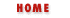### 8.2 Multidimensional Arrays

JavaScript does not support true multidimensional arrays, but it does allow you to approximate them quite nicely with arrays of arrays. To access a data element in an array of arrays, simply use the [] operator twice. For example, suppose the variable matrix is an array of arrays of numbers. Every element matrix[x] is an array of numbers. To access a particular number within this array you would write matrix[x][y].

Instead of using arrays of arrays, you can also use associative arrays to simulate multidimensional arrays. Because an associative array allows an arbitrary string as its index, it is easy to use them to simulate multidimensional arrays--i.e., to look up a value based on more than one index. You could use the following function, for example, to simulate reading a value from a three-dimensional array:

```function index3(arr, x, y, z)
{
return arr[x + "," + y + "," + z];
}
```

This example works because it combines the x, y, and z index values into a single, unique string that acts as a property name in the associative array (or object).Array ElementsArray/Object Dual Nature